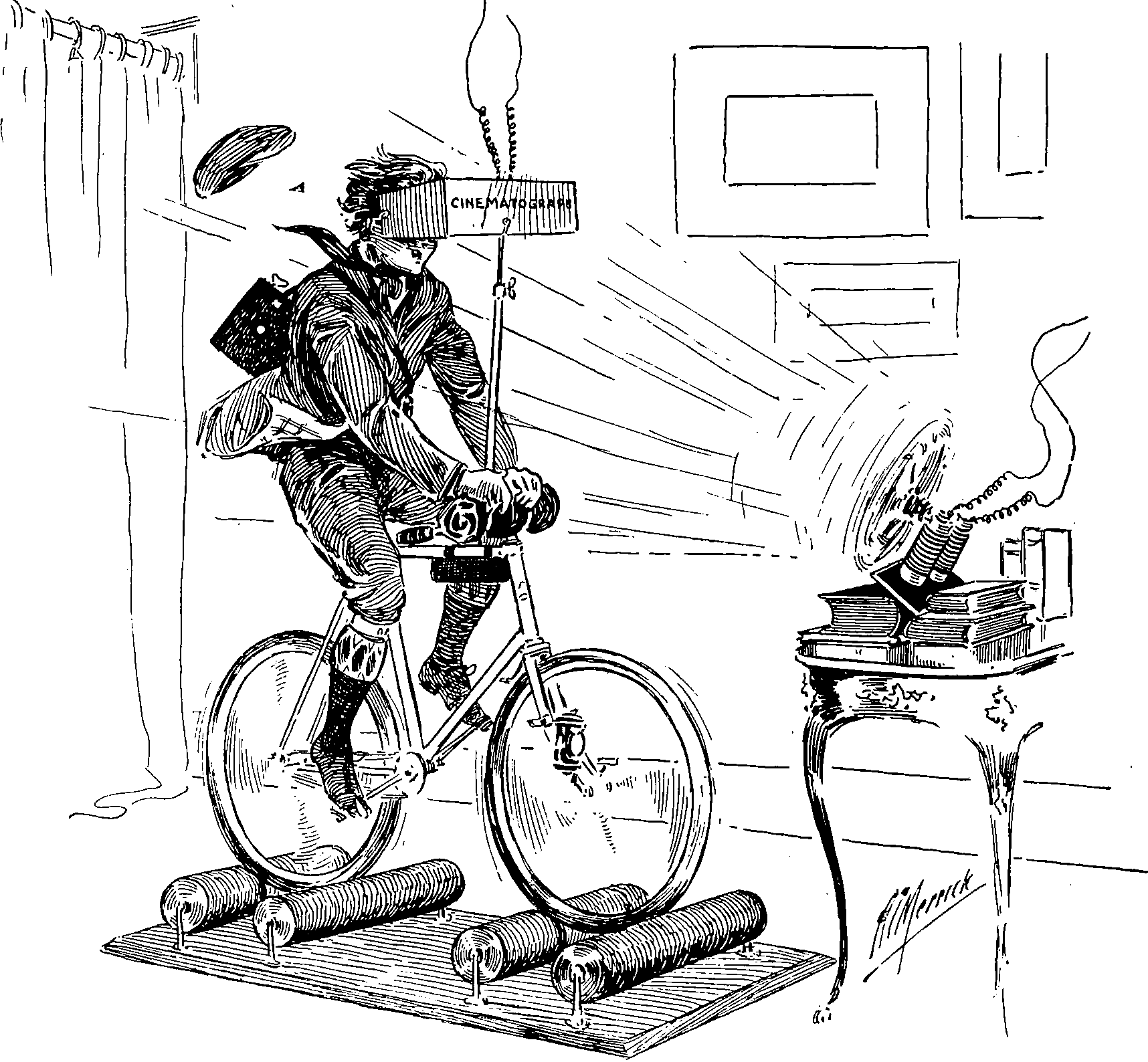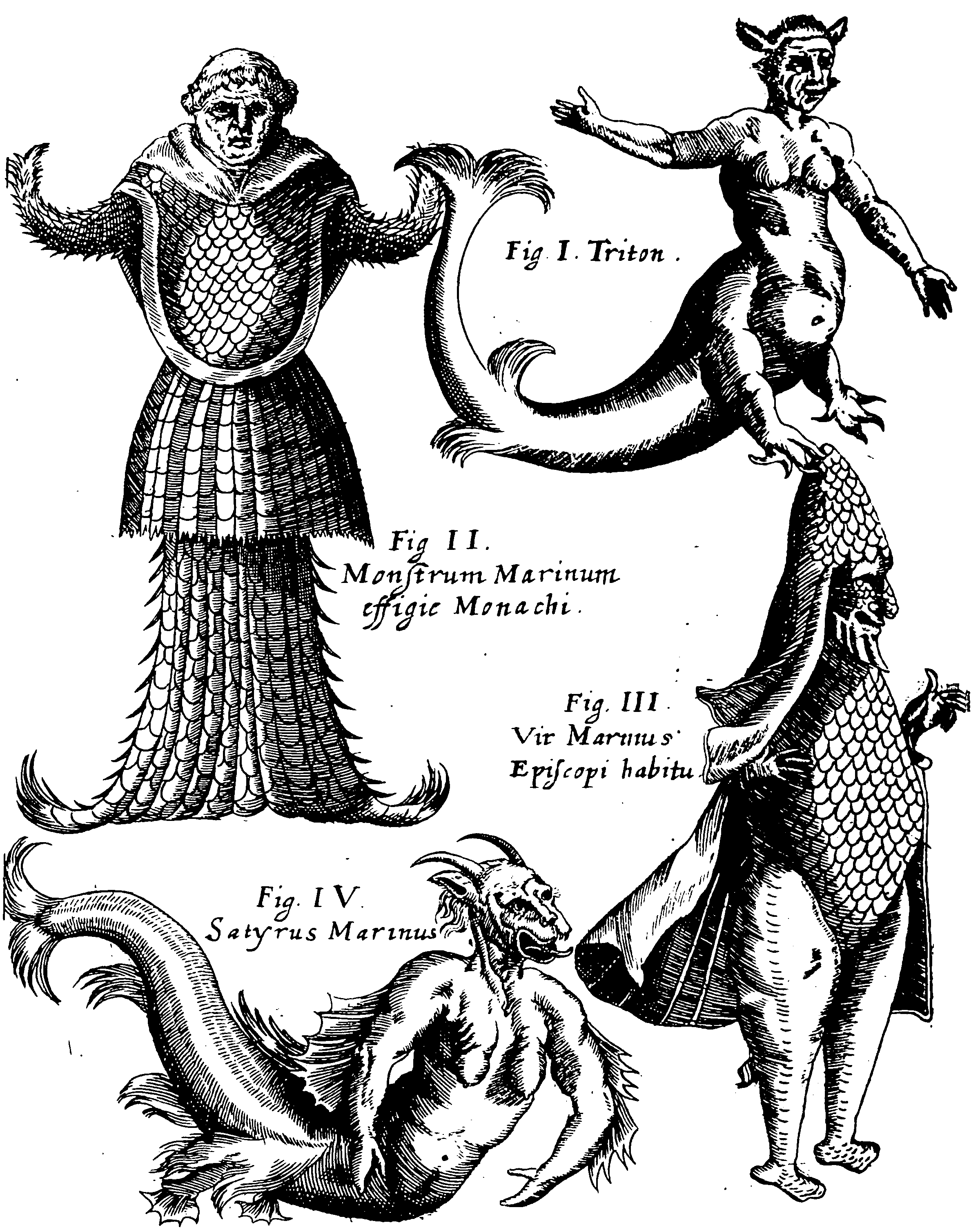# Kernel warpingA nonlinear way of transforming stationary kernels into non-stationary ones by transforming their inputs .

This is of interest in the context of composing kernels to have known desirable properties by known transforms, and also learning (somewhat) arbitrary transforms to attain stationarity.

## Stationary reducible kernelsThe main idea is to find a new feature space where stationarity or local stationarity can be achieved.

summarises:

We say that a nonstationary kernel $$K(\mathbf{x}, \mathbf{z})$$ is stationary reducible if there exist a bijective deformation $$\Phi$$ such that: $K(\mathbf{x}, \mathbf{z})=K_{S}^{*}(\mathbf{\Phi}(\mathbf{x})-\mathbf{\Phi}(\mathbf{z}))$ where $$K_{S}^{*}$$ is a stationary kernel.

## Classic deformations

### As a function of input

Invented apparently by and generalised in .

Let $$k_S$$ be some stationary kernel on $$\mathbb{R}^D.$$ Let $$\Sigma(\mathbf{x})$$ be a $$D \times D$$ matrix-valued function which is positive definite for all $$\mathbf{x},$$ and let $$\Sigma_{i} \triangleq \Sigma\left(\mathbf{x}_{i}\right) .$$ ) Then define $Q_{i j}=\left(\mathbf{x}_{i}-\mathbf{x}_{j}\right)^{\top}\left(\left(\Sigma_{i}+\Sigma_{j}\right) / 2\right)^{-1}\left(\mathbf{x}_{i}-\mathbf{x}_{j}\right)$ Then $k_{\mathrm{NS}}\left(\mathbf{x}_{i}, \mathbf{x}_{j}\right)=2^{D / 2}\left|\Sigma_{i}\right|^{1 / 4}\left|\Sigma_{j}\right|^{1 / 4}\left|\Sigma_{i}+\Sigma_{j}\right|^{-1 / 2} k_{\mathrm{S}}\left(\sqrt{Q_{i j}}\right)$ is a valid non-stationary covariance function.

Homework question: Is this a product of convolutional gaussian processes.

## Learning transformsWarning! Experimental comments system! If is does not work for you, let me know via the contact form.